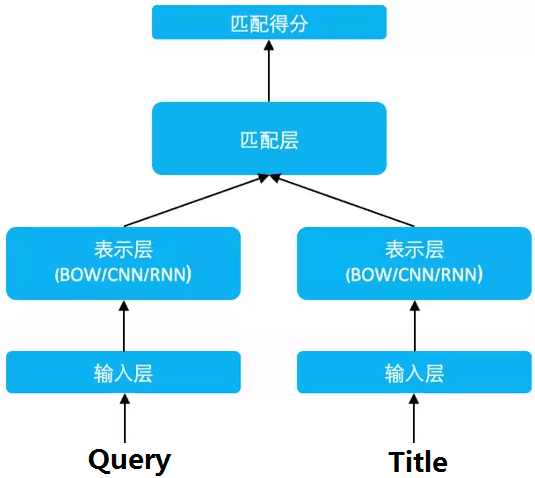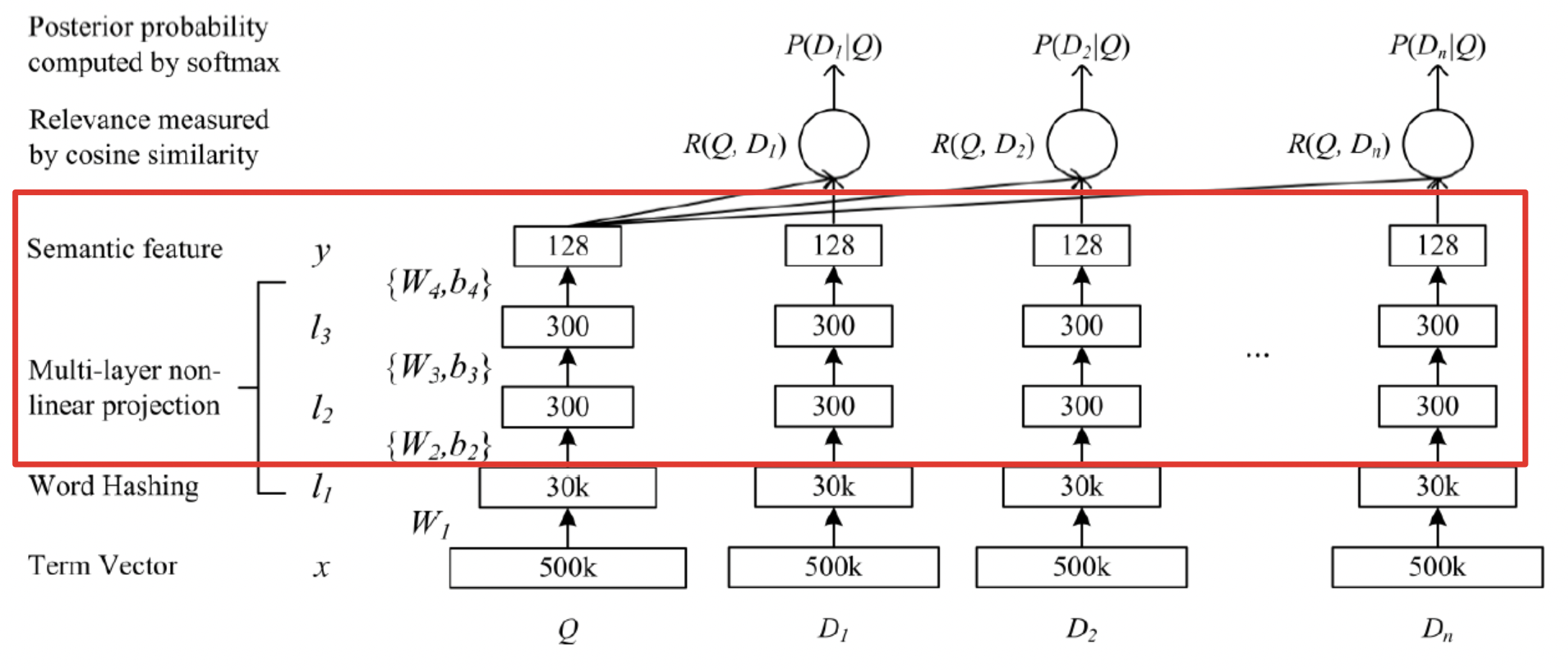# DSSM¶

## DSSM¶

DSSM（Deep Structured Semantic Models）的原理很简单，通过搜索引擎里 Query 和 Title 的海量的点击曝光日志，用 DNN 把 Query 和 Title 表达为低纬语义向量，并通过 cosine 距离来计算两个语义向量的距离，最终训练出语义相似度模型。该模型既可以用来预测两个句子的语义相似度，又可以获得某句子的低纬语义向量表达。

DSSM 从下往上可以分为三层结构：输入层、表示层、匹配层### 输入层¶

#### 英文¶

Letter-Bigram Letter-Trigram
word Size Token Size Collision Token Size Collision
40k 1107 18 10306 2
500k 1607 1192 30621 22

### 表示层¶

DSSM 的表示层采用 BOW（Bag of words）的方式，相当于把字向量的位置信息抛弃了，整个句子里的词都放在一个袋子里了，不分先后顺序。 紧接着是一个含有多个隐层的 DNN，如下图所示：$$W_{i}$$ 表示第 i 层的权值矩阵，$$b_{i}$$表示第 i 层的偏置项。则第一隐层向量 l2（300 维），第 二个隐层向量 l3（300 维），输出向量 y（128 维）,用数学公式可以分别表示为：

$l_{1}=W_{1}x$
$l_{i}=f(W_{i}l_{i-1}+b_{i}) ,i=2,...,N-1$
$y=f(W_{N}l_{N-1}+b_{N})$

$f(x)=\frac{1-e^{-2x}}{1+e^{-2x}}$

### 匹配层¶

Query 和 Doc 的语义相似性可以用这两个语义向量(128 维) 的 cosine 距离来表示：

$R(Q,D)=cosine(y_{Q},y_{D})=\frac{y_{Q}^Ty_{D}}{||y_{Q}|| ||y_{D}||}$

$P(D^{+}|Q)=\frac{exp(\gamma R(Q,D^{+}))}{\sum_{D^{'}\in D}exp(\gamma R(Q,D^{'}))}$

$L(\Lambda)=-log \prod_{(Q,D^{+})}P(D^{+}|Q)$

### 优缺点¶

• 优点：DSSM 用字向量作为输入既可以减少切词的依赖，又可以提高模型的泛化能力，因为每个汉字所能表达的语义是可以复用的。另一方面，传统的输入层是用 Embedding 的方式（如 Word2Vec 的词向量）或者主题模型的方式（如 LDA 的主题向量）来直接做词的映射，再把各个词的向量累加或者拼接起来，由于 Word2Vec 和 LDA 都是无监督的训练，这样会给整个模型引入误差，DSSM 采用统一的有监督训练，不需要在中间过程做无监督模型的映射，因此精准度会比较高。

• 缺点：上文提到 DSSM 采用词袋模型（BOW），因此丧失了语序信息和上下文信息。另一方面，DSSM 采用弱监督、端到端的模型，预测结果不可控。

## 参考文献¶

. Huang P S, He X, Gao J, et al. Learning deep structured semantic models for web search using clickthrough data[C]// ACM International Conference on Conference on Information & Knowledge Management. ACM, 2013:2333-2338.